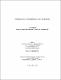## Investigations on some exponential congruences2016
##### Authors
Bose, Arnab
University of Lethbridge. Faculty of Arts and Science
##### Publisher
Lethbridge, Alta : University of Lethbridge, Dept. of Mathematics and Computer Science
##### Abstract
Selfridge asked for what positive integers a and b with a > b, does 2a — 2b divide na — nb for all n e N. The problem was solved by various people who showed that the above problem is true only for (a, b) e S, where S = {(2,1), (3,1), (4,2), (5,1), (5,3), (6,2), (7,3), (8,2), (8,4), (9,3), (14,2), (15,3), (16,4)}. In this thesis, we prove two generalizations of the above problem. Theorem. For a fixed positive integer m, na — nb = 0 (mod ma — mb) has a solution in (a, b) e N2 with a > b, for all integers n > m if and only ifm = 2 and (a, b) e S, where S is as given above. Zaharescu and Vajaitu considered a generalization of Selfridge’s problem in algebraic number fields. Our second result makes their theorem explicit and provides explicit bounds for the solutions. Next, we give a conditional resolution to a problem proposed by Ruderman which is related to Selfridge’s problem and also investigate some generalizations. Lastly, we use a particular case of the Schmidt Subspace Theorem and generalize a result proved by Bugeaud, Corvaja and Zannier .
##### Keywords
mathematics , number theory , Ruderman's problem , Schmidt Subspace Theorem , Selfridge's problem